# Double-track line

A 160 m long passenger train runs on a double-track line in one direction at a constant speed of 54 km/h, and a 240 m long express train in the opposite direction.

a) How fast is the express train if passing the passenger train driver for 6 s?
b) How long does the passenger train pass the express train driver?

v2 =  90 km/h
t2 =  4 s

### Step-by-step explanation: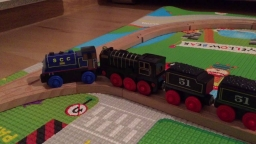Did you find an error or inaccuracy? Feel free to write us. Thank you!Tips to related online calculators
Do you have a linear equation or system of equations and looking for its solution? Or do you have a quadratic equation?
Do you want to convert length units?
Do you want to convert velocity (speed) units?
Do you want to convert time units like minutes to seconds?

## Related math problems and questions:

• Two trainsThe train runs at speed v1 = 72 km/h. The passenger, sitting in the train, observed that a train long l = 75m in 3 s passed on the other track in the opposite direction. Calculate the speed of this train.
• Two trainsThrough the bridge, long l = 240m, the train passes through the constant speed at time t1 = 21s. A train running along the traffic lights at the edge of the bridge passes the same speed at t2 = 9s. a) What speed v did the train go? b) How long did it take
• Double-track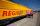On double-track express met with a freight train. Express travel at speed 72 km/h, a freight train at speed 36 km/h. After what time period will be from 9 km apart?
• Two trains meetFrom A started at 7:15 express train at speed 85 km/h to B. From B started passenger train at 8:30 in the direction to A and at speed 55 km/h. The distance A and B are 386 1/4 km. At what time and at what distance from B the two trains meet?
• Express train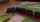International express train drove from Kosice to Teplice. In the first 279 km, the track was repaired, and therefore it was moving at a speed of 10km/h less than it was scheduled to drive. The rest of the 465 km trip has increased the speed by 8 km/h thanThe railroad runs parallel to the railway. The train travels at 36 km/h on track. In the opposite direction, the motorcycle rides at 90 km/h. The train passes in 3 seconds. How long is a train?
• Traffic collisionWhen investigating a traffic accident, it was found that the driver stopped the vehicle immediately after the accident by constant braking on a 150 m track in 15 seconds. Do you approve that the driver exceeded the permitted speed (50 km/h) in the village
• CarThe driver of a car is to get to 608 km distant city. From atlas found that 162 km will have to pass through the cities at average speed 48 km/h. Remainder of the journey pass outside the cities at average speed 116 km/h. Calculate how many hours it will
• Train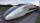A passenger train traveled for 2 hours 74 km. 3.1 hours after its departure started fast train and caught it on 186 km. How many km/h is different its average speeds?
• Reconstruction of the corridorCalculate how many minutes will be reduced to travel 187 km long railway corridor, where the maximum speed increases from 120 km/h to 160 km/h. Calculate how many minutes will shorten travel time, if we consider that the train must stop at 6 stations, eac
• The escalator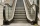I run up the escalator at a constant speed in the stairs' direction and write down the number of steps A we climbed. Then we turn around and run it at the same constant speed in the opposite direction and write down the number of steps B that I climbed. I
• Overtaking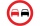On the direct road, the passenger car overtakes the slower bus by starting to overtake 20 meters from the bus and after passing it ahead of it again 20 meters away. The car overtakes at a steady speed of 72 km/h, the bus goes at a steady speed of 54 km/h.
• ICE train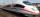German runways test a new ICE train between Munich and Berlin. The train runs to Berlin at a slow speed of 100 km/h. Back from Berlin goes faster. How quickly did the train have to go on a return trip so that the total average train speed for both journey
• Two vans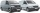At 8:30 a. M. , two vans leave at a parking area. They travel in opposite directions: one van travels at 48 km/h and the other at 62 km/h. At what time will the cars be 550 km apart?
• Train speed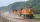The train speed is decreased during 50 sec from 72 km/h to 36 km/h. Assuming that the train movement is equally slowing, find the the acceleration and the distance that it travels at.
• TrainsFrom station 130 km away started passenger train and after 2.2 hours after the express train, which travels 37 km an hour more. Express train finish journey 7 minutes early. Calculate the average speed of this two trains.
• Sprinter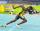Sprinter runs the relay 4 x 400 m to the handover at a speed of 42 km/h. A second runner is at the start of the handover area 20 m long and runs when it is the first sprinter at a distance of 10 m. Calculate the speed at which the second runner must run t